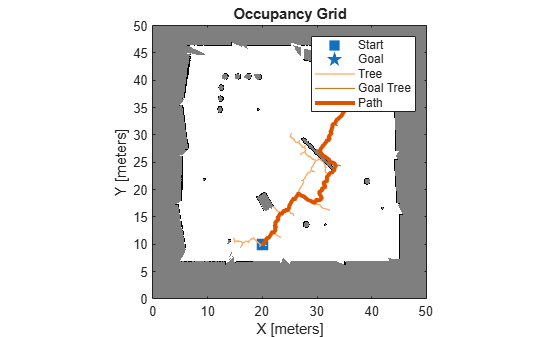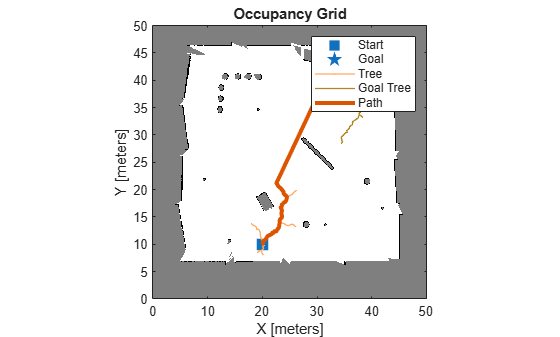# plan

Plan path between two states

## Syntax

``path = plan(planner,startState,goalState)``
``[path,solnInfo] = plan(planner,startState,goalState)``

## Description

````path = plan(planner,startState,goalState)` returns a bidirectional rapidly exploring random tree (RRT) path from the start state to the goal state as a `navPath` object.```

example

````[path,solnInfo] = plan(planner,startState,goalState)` also returns the solution information from path planning.```

## Examples

collapse all

Use the `plannerBiRRT` object to plan a path between two states in an environment with obstacles. Visualize the planned path with interpolated states.

Create a state space.

`ss = stateSpaceSE2;`

Create an `occupancyMap`-based state validator using the created state space.

`sv = validatorOccupancyMap(ss);`

Create an occupancy map from an example map and set map resolution as 10 cells per meter.

```load exampleMaps map = occupancyMap(ternaryMap,10);```

Assign the occupancy map to the state validator object. Specify the sampling distance interval.

```sv.Map = map; sv.ValidationDistance = 0.01;```

Update the state space bounds to be the same as the map limits.

`ss.StateBounds = [map.XWorldLimits; map.YWorldLimits; [-pi pi]];`

Create the path planner and increase the maximum connection distance.

```planner = plannerBiRRT(ss,sv); planner.MaxConnectionDistance = 0.3; % planner = plannerBiRRT(ss,sv,MaxConnectionDistance=0.3);```

Specify the start and goal states.

```start = [20 10 0]; goal = [40 40 0];```

Plan a path. Due to the randomness of the RRT algorithm, set the `rng` seed for repeatability.

```rng(100,'twister') [pthObj,solnInfo] = plan(planner,start,goal);```

Display the number of iterations taken for the tree to converge.

`fprintf("Number of iterations: %d\n",solnInfo.NumIterations)`
```Number of iterations: 346 ```

Visualize the results.

```show(map) hold on % Start tree expansion plot(solnInfo.StartTreeData(:,1),solnInfo.StartTreeData(:,2), ... '.-','color','b') % Goal tree expansion plot(solnInfo.GoalTreeData(:,1),solnInfo.GoalTreeData(:,2), ... '.-','color','g') % Draw path plot(pthObj.States(:,1),pthObj.States(:,2),'r-','LineWidth',2) hold off```Replan the path with the `EnableConnectHeuristic` property set to true.

```planner.EnableConnectHeuristic = true; [pthObj,solnInfo] = plan(planner,start,goal);```

Display the number of iterations taken for the tree to converge. Observe that the planner requires significantly fewer iterations compared to when the `EnableConnectHeuristic` property is set to false.

`fprintf("Number of iterations: %d\n",solnInfo.NumIterations)`
```Number of iterations: 192 ```

Visualize the results.

```figure show(map) hold on % Start tree expansion plot(solnInfo.StartTreeData(:,1),solnInfo.StartTreeData(:,2), ... '.-','color','b') % Goal tree expansion plot(solnInfo.GoalTreeData(:,1),solnInfo.GoalTreeData(:,2), ... '.-','color','g') % Draw path plot(pthObj.States(:,1),pthObj.States(:,2),'r-','LineWidth',2)```Load a 3-D occupancy map of a city block into the workspace. Specify the threshold to consider cells as obstacle-free.

```mapData = load("dMapCityBlock.mat"); omap = mapData.omap; omap.FreeThreshold = 0.5;```

Inflate the occupancy map to add a buffer zone for safe operation around the obstacles.

`inflate(omap,1)`

Create an SE(3) state space object with bounds for state variables.

`ss = stateSpaceSE3([0 220;0 220;0 100;inf inf;inf inf;inf inf;inf inf]);`

Create a 3-D occupancy map state validator using the created state space. Assign the occupancy map to the state validator object. Specify the sampling distance interval.

```sv = validatorOccupancyMap3D(ss, ... Map = omap, ... ValidationDistance = 0.1);```

Create a bidirectional RRT path planner with increased maximum connection distance and reduced maximum number of iterations. Set `EnableConnectHeuristic` property to true.

```planner = plannerBiRRT(ss,sv, ... MaxConnectionDistance = 50, ... MaxIterations = 1000, ... EnableConnectHeuristic = true);```

Specify start and goal poses.

```start = [40 180 25 0.7 0.2 0 0.1]; goal = [150 33 35 0.3 0 0.1 0.6];```

Configure the random number generator for repeatable result.

`rng(1,"twister");`

Plan the path.

`[pthObj,solnInfo] = plan(planner,start,goal);`

Visualize the planned path.

```show(omap) axis equal view([-10 55]) hold on % Start state scatter3(start(1,1),start(1,2),start(1,3),"g","filled") % Start tree expansion plot3(solnInfo.StartTreeData(:,1),solnInfo.StartTreeData(:,2), ... solnInfo.StartTreeData(:,3),".-",Color="g") % Goal state scatter3(goal(1,1),goal(1,2),goal(1,3),"y","filled") % Goal tree expansion plot3(solnInfo.GoalTreeData(:,1),solnInfo.GoalTreeData(:,2), ... solnInfo.GoalTreeData(:,3),".-",Color="y") % Path plot3(pthObj.States(:,1),pthObj.States(:,2),pthObj.States(:,3), ... "m-",LineWidth=2)```## Input Arguments

collapse all

Path planner, specified as a `plannerBiRRT` object.

Start state of the path, specified as an N-element real-valued vector. N is the number of dimensions in the state space.

Example: `[1 1 pi/6]`

Example: `[40 180 25 0.7 0.2 0 0.1]`

Data Types: `single` | `double`

Goal state of the path, specified as an N-element real-valued vector. N is the number of dimensions in the state space.

Example: `[2 2 pi/3]`

Example: `[150 33 35 0.3 0 0.1 0.6]`

Data Types: `single` | `double`

## Output Arguments

collapse all

Planned path information, returned as a `navPath` object.

Solution Information, returned as a structure. The structure contains these fields:

FieldDescription
`IsPathFound`Indicates whether a path is found. It returns `1` (`true`) if a path is found. Otherwise, it returns `0` (`false`).
`ExitFlag`

Indicates the termination cause of the planner, returned as:

• `1` — The planner reaches the goal.

• `2` — The planner reaches the maximum number of iterations.

• `3` — The planner reaches the maximum number of nodes.

`StartTreeNumNodes`Number of nodes in the start search tree when the planner terminates, excluding the root node.
`GoalTreeNumNodes`Number of nodes in the goal search tree when the planner terminates, excluding the root node.
`NumIterations`Number of combined iterations by both the start tree and goal tree.
`StartTreeData`Collection of explored states that reflect the status of the start search tree when the planner terminates. Note that `NaN` values are inserted as delimiters to separate each individual edge.
`GoalTreeData`Collection of explored states that reflect the status of the goal search tree when the planner terminates. Note that `NaN` values are inserted as delimiters to separate each individual edge.

Data Types: `structure`

## Version History

Introduced in R2021a